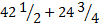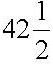# User ForumSubject :IMO    Class : Class 6

Class : Class 1

(B):  Cost of 1 kg rice = ₹ 15
Cost of 10 kg rice = ₹ (15 × 10) = ₹ 150
Cost of 1 kg sugar = ₹ 20
Cost of 15.04 kg sugar = ₹ (20 × 15.04)
= ₹ 300.80
Cost of 1 kg salt = ₹ 9
Cost of 5.06 kg salt = ₹ (9 × 5.06) = ₹ 45.54
Total cost = ₹ (150 + 300.80 + 45.54) = ₹ 496.34
Amount of money Chinmay gave to shopkeeper
= ₹ 1000
∴ Amount of money he get back
= ₹ (1000 - 496.34) = ₹ 503.66

Subject :IMO    Class : Class 4

Class : Class 1

(C):

1 kg = 1000g
Cost of 1 kg i.e 1000g oranges = Rs 120
Cost of 250g oranges = (120 / 1000) x 250 = Rs 30
Cost of 1kg  i.e 1000g apples = Rs 150
Cost of 500g apples = (150 / 1000) x 500 = Rs 75
Cost of 250g  oranges and 500g apples altogether = 30 + 75 = Rs 105

Subject :IMO    Class : Class 7

## Ans 1:

Class : Class 10

Subject :IMO    Class : Class 2

## Ans 1:

Class : Class 5

Subject :IMO    Class : Class 9

Subject :IMO    Class : Class 5

Class : Class 5

## Ans 2:

Class : Class 7

Subject :IMO    Class : Class 7

Class : Class 1

(C)

She will be facing North West after turning 45° anticlockwise. After turning 135° clockwise she will be facing East.
Finally after turning 90° clockwise she will be facing South.

Subject :IMO    Class : Class 7

Class : Class 1

(D): Quantity of juice Shivam purchased in two days == 85/2 + 99/4
= ( 170 + 99) / 4
= 269/4
= 67 1/4 litres
67 ¼ litres is approximately equal to 67 litres.

Subject :IMO    Class : Class 7

Class : Class 1

(D)

Number of fruits liked by A = 9, B =8, G = 8, M =10 and O = 7.
Answer-(D) B and G are liked by an equal number of students.

Subject :IMO    Class : Class 7